Next: Computing F: II Up: No Title Previous: The Fundamental Matrix

# Example 2 - General Motion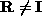:

• A point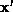corresponding toalways lies on its epipolar line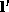, but in generaldoes not lie on.

• A general motion can be thought of as a pure translation of the optical centre, followed by a rotation of the image plane about the optical centre. The effects of the rotation can be ``undone'' by a projective transformation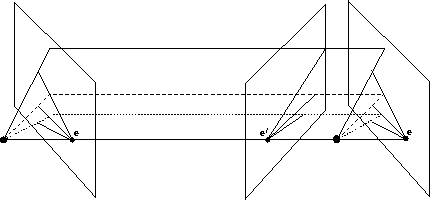Computing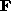: I Number of Correspondences Given perfect image points (no noise) in general position. Each point correspondence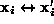generates one constraint on.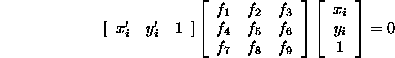This can be rearranged as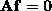where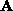is a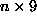measurement matrix, andis the fundamental matrix represented as a 9-vector.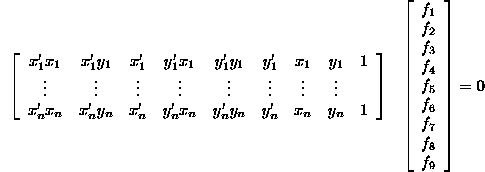• 8 or more points in general position: The dimension of the null-space ofis one., and consequently, is determined uniquely up to scale.

• 7 points in general position: The dimension of the null-space is two.

Suppose the 9-vectors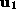and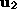, corresponding to the matrices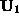and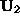respectively, span the null-space. Then, up to scale, there is a one-parameter family of matrices: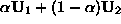. Imposing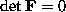which is a cubic in, and thus has either one or three real solutions.

Bob Fisher
Wed Apr 16 00:58:54 BST 1997